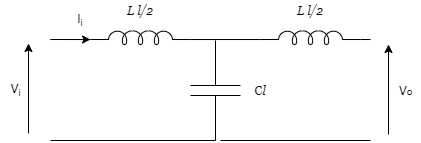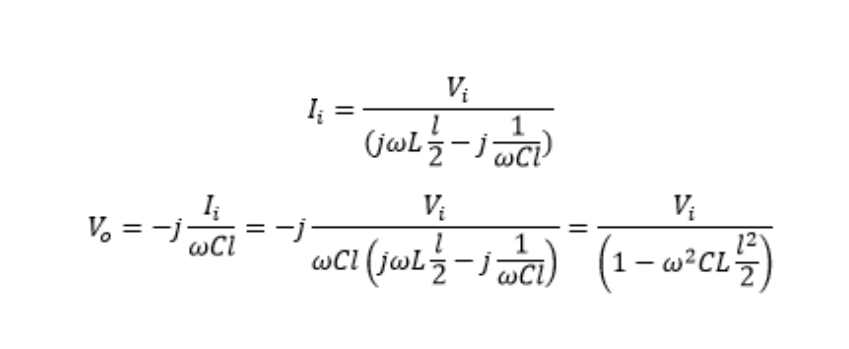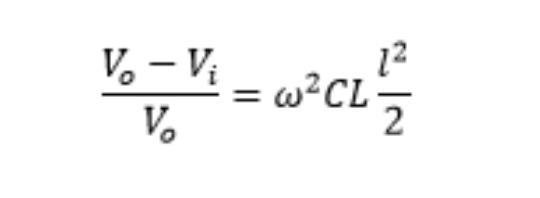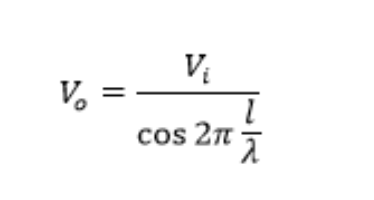Technical Article

# Understanding the Ferranti Effect in Transmission Lines

March 12, 2021 by Pietro Tumino

## This article presents an overview of the Ferranti effect, describing the causes and consequences of the phenomenon and highlighting which main power system parameters involved.

The Ferranti Effect is a voltage increase in the receiving end of an electrical transmission line when it is operated in a no-load, or low-load, condition. This results in a receiving end voltage value higher than the sending point.

This phenomenon was discovered by electrical engineer Sebastian Ziani de Ferranti. In 1887, he first noted a voltage increase in certain points of a London power system.

The effect is caused by the interaction between the inductance and capacitance of the line.

When the power line is operated at no-load, or low load conditions,  the voltage at the receiving end can be higher than the input voltage.If this voltage exceeds the rated value of the line, it can result in dangerous situations and cause stress to the cables and components.

The classic T-scheme shown in the figure below helps to explain how the Ferranti effect occurs in an electric transmission line. Here, we assume that the resistive behavior of the line is negligible.T Scheme of a transmission line.

Where:

• L is the longitudinal inductance of the line [H/km]
• l is the length of the line [km]
• C is the transversal capacitance of the line [F/km]
• Vi is the voltage at the input of the line
• Ii is the current at the input of the line
• Vo is the voltage at the output of the line

The below equation assumes the line is in a “no-load” condition (open circuit) and applies the Kirchoff principle to the circuit above:From the circuit model, due to the no-load conditions, it is evident that the output voltage is the voltage on the capacitance. We’ll see that the transversal capacitance of the line plays a key role in the Ferranti effect.

From the equations above it is possible to notice that the output voltage Vo is higher than the input voltage Vi  and in particular calculating the difference between them, referring it to the output voltage, we have:Therefore, it’s evident that the voltage difference is proportional to:

• The square of the frequency of the power system (indeed =2f)
• The product of line inductance and capacitance
• The square of the line length

These observations bring us to several conclusions that are worth mentioning:

Transmission lines in power systems that operate at a higher frequency are more likely to be affected by the Ferranti Effect. For example, considering two identical electrical lines operated at the same voltage but at different frequencies, the one operated at a higher frequency must be shorter in order to avoid undesired and dangerous voltage increase at the receiving end.

With cable lines, the Ferranti Effect will be more pronounced, because the typical values of the service inductance in a cable are approximately 0.5 to 0.7 times the inductance of an overhead line. However, the values of the capacitance are about 20 to 60 times higher. Therefore, with a cable line, the product of the line inductance and capacitance can be about 10 to 30 times higher.

The length of the line is crucial. However, the length becomes even more important when the line length gets closer to /4. Considering the trigonometric notation of the equations of the transmission lines, at no-load condition, it is possible to demonstrate that:So, for 0l/4 (so for 0l1500 km at 50 Hz) we have 02l//2, therefore the term at the denominator is between 1 and 0. As the line length approaches  /4the voltage at the receiving end of the line tends to be infinite.

As mentioned above, the main reason for the Ferranti Effect is the interaction between the capacitance and inductance of the line.

Overall, the Ferranti Effect is well known and should be taken into consideration in the design of a power distribution system in order to avoid unexpected voltage increases that can create failures and dangerous situations.

To avoid the Ferranti Effect, we need to limit the maximum length of the electrical transmission lines. That is why typical power transmission lines don’t exceed 600-700 km at 50 Hz, (or 500-600 km at 60Hz).

By reducing the length of the line in order to minimize this phenomenon, the inductance and capacitance of the line can create a resonance situation, due to the intrinsic constructive properties of the line itself. To avoid the Ferranti Effect, the common solution is to install an additional reactor (basically an inductance). This compensates for the transversal capacitance of the line and drastically reduces this phenomenon.

The reality of these issues can become much more complex than the explanations offered above. One must think of any possible losses, instead of assuming the “ideal” case of the line with a no-load condition.

#### NOTE: �� is the wavelength (��=v/f) defined as the ratio between the velocity of the wave propagation and the frequency